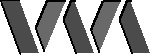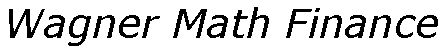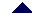Home Products Consulting People Clients Papers News Contact UsProductsRetirement PlanningRSPM-V OptimizationM-V OptimizerFAQUser's GuideTech SupportMVO LibraryStyle AnalysisWAStylerSA Library

# Mean-Variance Optimization Frequently Asked Questions

What is a positive semi-definite matrix?

Here is the technical definition: a matrix M is positive definite if for all non-zero vectors x, the quantity xTMx is positive. (The notation xT indicates the transpose of the vector x.) The matrix M is positive semi-definite if xTMx is non-negative for all non-zero vectors x. To put this concept into context, we are concerned with correlation (R = rij) and covariance matrices (S = sij) of assets in our universe. By definition, these matrices are symmetric and related to each other in the following way: sij = rijvivj , where the asset volatility vi is the square root of sii. If the vector x represents the allocation of assets in a feasible portfolio, we can calculate the volatility of the portfolio as the square root of xTSx. For this to be well-defined for all feasible portfolios, we require that xTSx be at least non-negative.Why isn't my correlation matrix positive semi-definite?

This can be caused in several ways - a non-exhaustive list follows. The most common reason is that you used fewer dates than assets when you calculated your correlation matrix. This leads to a correlation matrix that is theoretically singular; i.e., its determinant is zero. (A necessary condition that a matrix is positive semi-definite is that its determinant is non-negative, but this is not sufficient; a more thorough test is performed within M-V Optimizer and WAoptimize.) This in itself is not disallowed (although it has implications regarding the uniqueness of the risk-free portfolio), but it makes your matrix more susceptible to being detected as non-positive semi-definite. Computers have finite precision, and calculations which involve adding and subtracting a lot of small numbers (as is the case when a determinant is computed) are prone to round-off error. This is the likely culprit, but it isn't the only one. You can also achieve this effect if you have a pair of assets that are almost perfectly correlated, or you have an asset with volatility very close to zero. If you entered your correlation matrix manually, you may have entered a few values incorrectly or your correlation matrix has insufficient precision (e.g., the values all have three place accuracy, and this loss of precision is sufficient to make the matrix non-positive semi-definite). In rare cases you have a complex combination of several of these effects.What can I do to make my correlation matrix positive semi-definite?

Sophisticated techniques involve using maximum likelihood estimation or principal components analysis methods to estimate the "closest" positive definite matrix to your non-positive semi-definite matrix. This is outside the scope of M-V Optimizer and WAoptimize, and probably a little bit beyond the capabilities of most individual users. We suggest the following general purpose approach. The basic idea is to perturb your matrix just enough to overcome the round-off and precision problems that are triggering the error report. Working with your correlation matrix, multiply the off-diagonal entries in the matrix by a number very close to 1 (say 0.9999). If this still fails, try 0.9995, 0.999, 0.995, 0.99, and so forth, until you find a value that works. The closer the multiplier is to 1, the less you disturb the basic correlation structure of your matrix. This works almost all the time as long as you started with a matrix that was generated in a reasonable way; i.e., this won't work with randomly generated matrices. The spreadsheet capabilities of Microsoft Excel are ideal for setting up such a procedure and systematically trying your multipliers. The latest release of M-V Optimizer has a version of this technique built-in (although it stops its attempts at 0.995 - we feel deviations beyond this point should be made with full knowledge by the user). For previous purchasers, contact us and we will be happy to provide you with a patch via e-mail.How much time series return data should I use?

Most users use M-V Optimizer and WAoptimize to help them rebalance their portfolio on an annual basis. Assuming this long-term investment assumption, users seek to find asset statistics (return and volatility) that approximate their long-term values. It would seem prudent to select a range of recent years that covers not only the recent bull market but also bear markets in the not-so-distant past. For example, a recent major bear market occurred in 1981-82, and a recent major crash (in percentage terms) occurred in October 1987. This would imply that 10 to 20 years worth of data would be a reasonable collection.Where can I get time series return data?

Wagner Associates does not supply return data, nor do we recommend any particular data source. We communicate with current users from time-to-time, and they relay to us the data sources they have found useful in their investment planning. We reproduce this list here solely for transfer of information purposes. If you know of a data source and would like to share it with other users, please contact us and we will be happy to update this list with your information:

• Morningstar
• Worden Brothers Telechart 2000
• Steele's Mutual Fund Expert, Pro Plus edition
• Yahoo! Finance
• RussellCan I use price data instead of return data?

You will need to convert the prices into returns.What do I do if I have different amounts of data for different assets?

M-V Optimizer replaces missing data values with zero. If you have a cell here or there that is empty, then this should not be a big deal. However, if you have 20 dates of data for asset A and 10 dates of data for asset B, then assuming the missing 10 dates of data for asset B are zero will severely bias your calculations. You have two simple choices for how to handle this situation: a) use only the number of dates that all assets have in common and use M-V Optimizer's or Excel's built-in functionality for calculating the statistics, or 2) use only the number of dates that each pair of assets have in common and manually build (i.e., use Excel's CORREL function) the correlation matrix one entry at a time.How do I print the M-V Optimizer or WAoptimize sheets to a black and white printer?

In Excel, go to your "File" pulldown and select "Page Setup". This should give you a dialog with several tabs. Go to the "Sheet" tab and check the box next to "Black and White". You will need to do this for each sheet you want to print. You need only do this once if you now save your workbook.What do the error codes mean in M-V Optimizer and WAoptimize?

M-V Optimizer and WAoptimize perform many internal tests. They check the input data as well as test the calculations performed inside the optimizer for consistency. There are many potential error codes that can be produced, most of which will be meaningful only to the developers of the software. If you receive an error code and cannot reasonably determine its source, then please contact technical support at Wagner Associates (MVOSupport@pa.wagner.com). In the vast majority of cases, the user is the source of the error. But as part of our continuing improvement program, our interest is in diagnosing and correcting any potential problem in the software. Some of the most common error codes are as follows:Why does M-V Optimizer return Error -2001 when I click OPTIMIZE PORTFOLIO?

Here are the known causes for Error -2001:

A) You did not reboot after installing M-V Optimizer.
Corrective action: reboot, then try again.

B) The required DLLs were installed to a network drive.
Corrective action: reinstall the software, accepting the default location for the DLLs.

C) The required DLLs were installed to different directories.
Corrective action: reinstall the software, accepting the default location for the DLLs. Verify that Crp9516a.dll, Cryp95a.dll, and MVOptim.dll all are in the Windows System directory.

D) You did not have permission to add files to the Windows System directory, or you did not have permission to add drivers and services to a Windows NT platform.
Corrective action: have someone with full permissions reinstall the software.

E) Windows was re-installed, and the Windows System directory is not in the same place as before, or the Windows NT drivers and services required by the licensing system did not survive the re-install.
Corrective action: have someone with full permissions reinstall the software.

Make a copy of MVOptimizer.xls before re-installing, if you don't want to retype any of your input data.I paid for a copy of M-V Optimizer, obtained a Site Key, and have been using it on a daily basis with no problems. When I tried to run an Optimize Portfolio today, I was told that my free trial had expired and I need to purchase a license. Why did this happen, and how can I prevent it from happening again?

Your M-V Optimizer license depends upon certain files remaining exactly where they were installed. It's possible that a Windows re-install, or a disk defragmenting utility has moved or corrupted these files. In particular, if you use the Speed Disk defragmenting utility from Symantec's Norton Utilities, you could damage the licensing files, causing an interruption of your license.

Before you defragment your hard drive with the Norton Utilities Speed Disk, you need to change its settings to avoid damaging the license files.

1. Open Speed Disk and choose File->Options->Customize->Unmovable Files.
2. Specify that *.ENT, *.KEY, and *.RST files cannot be moved.
NOTE: Speed Disk's default settings allow system files to be moved, but moving any of these 3 file types can disrupt your license.
3. Choose File->Options->Optimization->Save to save these settings.

It now should be safe to use Speed Disk without disrupting your license.Can I calculate tracking portfolios using M-V Optimizer or WAoptimize?

The classic tracking portfolio problem has the following form:

n = number of assets in the universe
x0 = benchmark portfolio weights (n by 1 vector)
x = tracking portfolio weights (n by 1 vector)
a = expected returns (n by 1 vector)
S = covariance matrix (n by n symmetric positive definite matrix)

Objective: determine x which minimizes the tracking index

(x - x0)TS(x - x0)
while maximizing the marginal return
aT(x - x0)

You can solve this problem using the MVO library by setting up the following problem:

n´ = n + 1 = number of assets in the universe
x´ = portfolio weights (n´ by 1 vector)
a´ = expected returns (n´ by 1 vector)
S´ = covariance matrix (n´ by n´ symmetric positive definite matrix)

where

x´ = [-1 ,   x]
a´ = [aTx0 ,   a]
S´ = [x0TSx0    x0TS ,   Sx0T    S]

Objective: determine which minimizes the squared risk

TS´x´
while maximizing the return
T

Note that

TS´x´ = (x - x0)TS(x - x0)

and

Tx´ = aT(x - x0)

To set this up using the MVO library, simply fix the holdings of the "additional asset" to -1 and build the return and covariance matrices as illustrated above.

To set this up using WAoptimize or M-V Optimizer, set the minimum allocation and maximum allocation of the "additional asset" to -1. Set the return of the "additional asset" to aTx0. Assume that the original correlation matrix is Y and the volatilities are given by the n by 1 vector s. Thus, the (i,j) entry of S is equal to the (i,j) entry of Y multiplied by sisj, or S = diag(s)Ydiag(s). (diag(s) is an n by n diagonal matrix with the volatilities on the diagonal.) The volatility, sa, of the "additional asset" is then given by the square root of x0TSx0 = x0Tdiag(s)Ydiag(s)x0 . The new correlation matrix has the form

Y´ = [1    (1/sa)x0Tdiag(s)Y ,   Ydiag(s)x0(1/sa)    Y]